• 今天小编就为大家分享一篇关于PyTorch 自动求导机制详解，具有很好的参考价值，希望对大家有所帮助。一起跟随小编过来看看吧

pytorch自动求导

学习过程中参考动手学深度学习pytorch版

基本概念

在调用y.backward()时，如果张量y是一个标量则不需要传入任何参数，如果不是标量则需要传入一个与y同形的张量，这里我的理解是其实和矩阵求导一样也就是点对点求导，如果对不上那就没办法进行求导，因此需要一个同形张量来完成一个点对点的求导操作。

print(x)

y = x + 2
print(y)

a = torch.randn(2, 2) # 不指定的情况下默认 requires_grad = False
a = ((a * 3) / (a - 1))
b = (a * a).sum()

输出：

False
True
<SumBackward0 object at 0x00000203BCE72160>

计算实例

print(x.is_leaf, y.is_leaf)
# True  False

为了体现链式求导的法则，当然也有复杂一些的操作，比如下面的先乘积在求均值的操作求导中：

z = y * y * 3
out = z.mean() #标量
print(z, out)

输出：

tensor([[27., 27.],

这里再来一个反向传播感受一下标量的求导不需要给.backward()传入任何参数：

#直接利用反向传播的方法
out.backward() # 等价于 out.backward(torch.tensor(1.))

输出：

tensor([[4.5000, 4.5000],
[4.5000, 4.5000]])

解释：
要计算的是out关于x的梯度 d ( o u t ) d x \frac{d(out)}{dx} ，我们令out o o , 因为
o = 1 4 ∑ i = 1 4 z i = 1 4 ∑ i = 1 4 3 ( x i + 2 ) 2 o=\frac14\sum_{i=1}^4z_i=\frac14\sum_{i=1}^43(x_i+2)^2
所以
∂ o ∂ x i ∣ x i = 1 = 9 2 = 4.5 \frac{\partial{o}}{\partial{x_i}}\bigr\rvert_{x_i=1}=\frac{9}{2}=4.5
所以上面的输出没有问题。在数学上矩阵的链式求导可以用雅可比矩阵和一个向量来表示，有兴趣可以查阅原书，我的公式打得还不熟。

下面来一个更复杂些的反向传播操作：

#梯度都会累加之前的梯度，所以一般在反向传播之前需把梯度清零。
out2 = x.sum()
out2.backward()
#先清零梯度在进行反向传播，不在累加
out3 = x.sum()
out3.backward()

为了避免梯度在求导过程中出现矩阵相乘或者维度不一致的问题，所以我们不允许张量对张量求导，只允许标量对张量求导，求导结果是和自变量同形的张量。所以必要时我们要把张量通过将所有张量的元素加权求和的方式转换为标量，举个例子，假设y由自变量x计算而来，w是和y同形的张量，则y.backward(w)的含义是：先计算l = torch.sum(y * w)，则l是个标量，然后求l对自变量x的导数。例如：

#不允许张量对张量求导，只允许标量对张量求导，求导结果是和自变量同形的张量
x = torch.tensor([1.0, 2.0, 3.0, 4.0], requires_grad=True)
y = 2 * x
print(y)
z = y.view(2, 2)
print(z)

#现在 z 不是一个标量，所以在调用backward时需要传入一个和z同形的权重向量进行加权求和得到一个标量。
v = torch.tensor([[1.0, 0.1], [0.01, 0.001]], dtype=torch.float)#z同形的权重向量
z.backward(v)

输出：

tensor([[2., 4.],
tensor([2.0000, 0.2000, 0.0200, 0.0020])

#梯度追踪
y1 = x ** 2
y2 = x ** 3
y3 = y1 + y2

输出：

True
tensor(1.) False

y3.backward()#反向传播

print(x.data) # 还是一个tensor

y = 2 * x
x.data *= 100 # 只改变了值，不会记录在计算图，所以不会影响梯度传播

y.backward()
print(x) # 更改data的值也会影响tensor的值

输出：

tensor([1.])
False
tensor([2.])
算图，所以不会影响梯度传播

y.backward()
print(x) # 更改data的值也会影响tensor的值

输出：

tensor([1.])
False
tensor([2.])

展开全文pytorch 神经网络 深度学习
• 自动求导机制 在即时执行模式下，Tensorflow引入tf.GradientTape()这个“求导记录器”来实现自动求导。 计算函数y(x)=x^2在x = 3时的导数： import tensorflow as tf #定义变量 x = tf.Variable(initial_value = ...

自动求导机制

计算函数y(x)=x^2在x = 3时的导数：

import tensorflow as tf
#定义变量
x = tf.Variable(initial_value = 3.)

#y = x^2
y = tf.square(x)
#计算y关于x的导数(斜率，梯度)

输出：

[<tf.Tensor: shape=(), dtype=float32, numpy=9.0>, <tf.Tensor: shape=(), dtype=float32, numpy=6.0>]
展开全文tensorflow
• 自动求导机制

2019-08-14 14:14:59

从后向中排除子图

如果有一个单一的输入操作需要梯度，它的输出也需要梯度。

相反，只有所有输入都不需要梯度，输出才不需要。如果其中所有的变量都不需要梯度进行，后向计算不会在子图中执行。

>>> x = Variable(torch.randn(5, 5))
>>> y = Variable(torch.randn(5, 5))
>>> z = Variable(torch.randn(5, 5), requires_grad=True)
>>> a = x + y
False
>>> b = a + z
True

model = torchvision.models.resnet18(pretrained=True)
for param in model.parameters():
# Replace the last fully-connected layer
# Parameters of newly constructed modules have requires_grad=True by default
model.fc = nn.Linear(512, 100)

# Optimize only the classifier
optimizer = optim.SGD(model.fc.parameters(), lr=1e-2, momentum=0.9)
展开全文自动求导机制
• 点击上方“AI公园”，关注公众号，选择加“星标“或“置顶”作者：Vaibhav Kumar编译：ronghuaiyang导读这篇文章详细解析了PyTorch的自动求导机制...

点击上方“AI公园”，关注公众号，选择加“星标“或“置顶”

者：Vaibhav Kumar

编译：ronghuaiyang

导读

这篇文章详细解析了PyTorch的自动求导机制，让你了解PyTorch的核心魔法。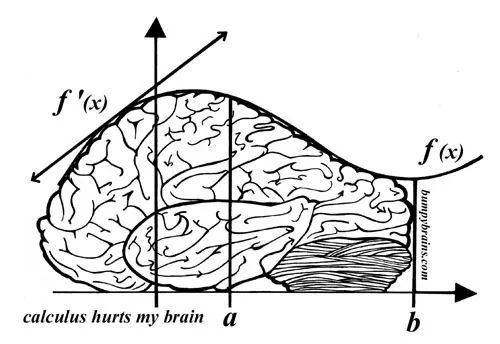在这个过程中，它从不显式地构造整个雅可比矩阵。 直接计算JVP通常更简单、更有效。

我们都同意，当涉及到大型神经网络时，我们都不擅长微积分。通过显式求解数学方程来计算这样大的复合函数的梯度是不现实的，特别是这些曲线存在于大量的维数中，是无法理解的。

要处理14维空间中的超平面，想象一个三维空间，大声地对自己说“14”。每个人都这么做——Geoffrey Hinton

PyTorch基础

在进一步讨论之前，我们需要了解一些基本的PyTorch概念。

import torch
import numpy as np

x = torch.randn(2, 2, requires_grad = True)

# From numpy
x = np.array([1., 2., 3.]) #Only Tensors of floating point dtype can require gradients
x = torch.from_numpy(x)
# _ above makes the change in-place (its a common pytorch thing)

创建启用梯度的张量的各种方法的代码

注意：根据PyTorch的设计，梯度只能计算浮点张量，这就是为什么我创建了一个浮点类型的numpy数组，然后将它设置为启用梯度的PyTorch张量。

神经网络和反向传播

神经网络只不过是经过精心调整(训练)以输出所需结果的复合数学函数。调整或训练是通过一种称为反向传播的出色算法完成的。反向传播用来计算相对于输入权值的损失梯度，以便以后更新权值，最终减少损失。

在某种程度上，反向传播只是链式法则的一个花哨的名字—— Jeremy Howard

创建和训练神经网络包括以下基本步骤：

1. 定义体系结构

2. 使用输入数据在体系结构上向前传播

3. 计算损失

4. 反向传播，计算每个权重的梯度

5. 使用学习率更新权重

损失变化引起的输入权值的微小变化称为该权值的梯度，并使用反向传播计算。然后使用梯度来更新权值，使用学习率来整体减少损失并训练神经网络。

这是以迭代的方式完成的。对于每个迭代，都要计算几个梯度，并为存储这些梯度函数构建一个称为计算图的东西。PyTorch通过构建一个动态计算图(DCG)来实现这一点。此图在每次迭代中从头构建，为梯度计算提供了最大的灵活性。例如，对于前向操作(函数)Mul ，向后操作函数MulBackward被动态集成到后向图中以计算梯度。

动态计算图

一个简单的DCG用于两个张量的乘法会是这样的：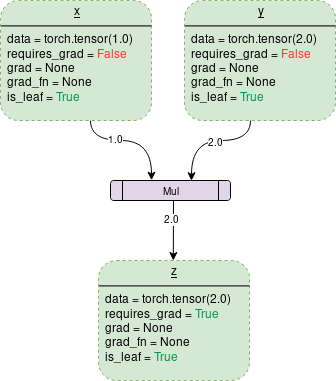图中的每个点轮廓框是一个变量，紫色矩形框是一个操作。

每个变量对象都有几个成员，其中一些成员是：

Data：它是一个变量持有的数据。x持有一个1x1张量，其值等于1.0，而y持有2.0。z持有两个的乘积，即2.0。

is_leaf：如果：

1. 它被一些函数显式地初始化，比如x = torch.tensor(1.0)x = torch.randn(1, 1)(基本上是本文开头讨论的所有张量初始化方法)。

3. 它是通过对某个张量调用.detach()方法创建的。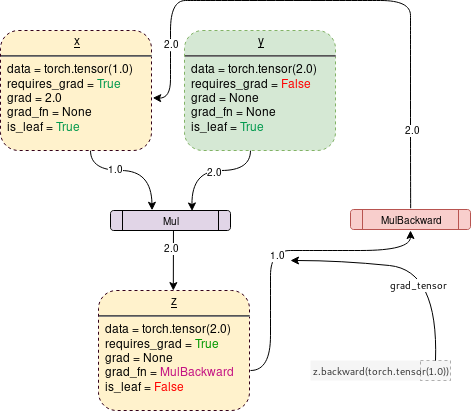在PyTorch下生成上图的代码是：

Backward()函数

让我们分析以下代码：

import torch
# Creating the graph
x = torch.tensor(1.0, requires_grad = True)
z = x ** 3
print(x.grad.data) #Prints '3' which is dz/dx

需要注意的一件重要事情是，当调用z.backward()时，一个张量会自动传递为z.backward(torch.tensor(1.0))torch.tensor(1.0)是用来终止链式法则梯度乘法的外部梯度。这个外部梯度作为输入传递给MulBackward函数，以进一步计算x的梯度。传递到.backward()中的张量的维数必须与正在计算梯度的张量的维数相同。例如，如果梯度支持张量x和y如下：

x = torch.tensor([0.0, 2.0, 8.0], requires_grad = True)
y = torch.tensor([5.0 , 1.0 , 7.0], requires_grad = True)
z = x * y

然后，要计算z关于x或者y的梯度，需要将一个外部梯度传递给z.backward()函数，如下所示：

z.backward(torch.FloatTensor([1.0, 1.0, 1.0])

z.backward() 会给出 RuntimeError: grad can be implicitly created only for scalar outputs

反向函数传递的张量就像梯度加权输出的权值。从数学上讲，这是一个向量乘以非标量张量的雅可比矩阵(本文将进一步讨论)，因此它几乎总是一个维度的单位张量，与 backward张量相同，除非需要计算加权输出。

数学—雅克比矩阵和向量

注意：在这个过程中，PyTorch从不显式地构造整个雅可比矩阵。直接计算JVP (Jacobian vector product)通常更简单、更有效。

如果一个向量X = [x1, x2，…xn]通过f(X) = [f1, f2，…fn]来计算其他向量，则雅可比矩阵(J)包含以下所有偏导组合：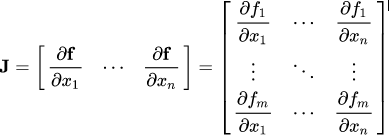雅克比矩阵

上面的矩阵表示f(X)相对于X的梯度。

假设一个启用PyTorch梯度的张量X

X = [x1,x2,…,xn](假设这是某个机器学习模型的权值)

X经过一些运算形成一个向量Y

Y = f(X) = [y1, y2，…,ym]

然后使用Y计算标量损失l。假设向量v恰好是标量损失l关于向量Y的梯度，如下：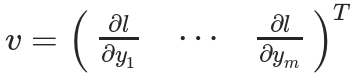为了得到损失的梯度l关于权重X的梯度，雅可比矩阵J是向量乘以向量v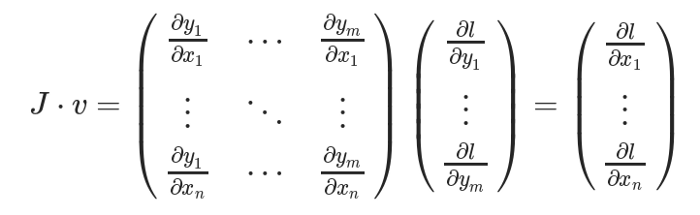这种计算雅可比矩阵并将其与向量v相乘的方法使PyTorch能够轻松地为非标量输出提供外部梯度。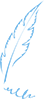— END—请长按或扫描二维码关注本公众号

喜欢的话，请给我个好看吧展开全文• PyTorch便捷的愿意之一——自动求导机制，以及其简单应用：Logistic回归pytorch 深度学习 机器学习 python
• pytorch：自动求导机制

千次阅读 2018-08-14 23:21:43pytorch 自动求导 autograd
• 这里写自定义目录标题pytorch自动求导机制——代码实现代码实现 pytorch自动求导机制——代码实现 代码实现 import torch import torch.nn as nn import numpy as np #########————————定义模型————...深度学习
• 基于这个思路，目前主流 AI 框架中有两种完全不同的自动求导机制： 基于对偶图变换的自动求导机制 基于 reverse mode 的自动求导机制 1.1 基于对偶图的自动求导机制 基于对偶图的自动求导机制的实现思路是，首先通过...python java 编程语言 人工智能 深度学习
• 文章目录PyTorch的计算图和自动求导机制自动求导机制简介自动求导机制实例梯度函数的使用计算图构建的启用和禁用 PyTorch的计算图和自动求导机制 自动求导机制简介 PyTorch会根据计算过程自动生成动态图，然后根据...python 深度学习 pytorch

千次阅读 2018-04-27 10:05:39pytorch
• 基于pytorch自动求导机制的牛顿迭代法实现框架，求解的函数可自行替换为任意函数
• Pytorch 自动求导机制 文章目录Pytorch 自动求导机制1. 简单的自动求导（输出是1维）2. 多维数组的自动求导（输出是多维）3. 多次自动求导 自动求导是 PyTorch 中非常重要的特性，能够让我们避免手动去计算非常复杂...python
• TensorFlow提供了强大的自动求导机制来计算导数。以下代码展示了如何使用tf.GradientTape(）方法计算函数的导数 # TensorFlow 提供了强大的自动求导机制来计算导数 import tensorflow as tf # 变量 # 变量与普通...
• Ps：在深度理解自动求导机制时，非常幸运一开始看了ronghuaiyang大神翻译的这篇博客https://blog.csdn.net/u011984148/article/details/99670194从而整体思路没有出现偏差，但是由于本人水平不够的原因好多地方都是...autograd
• 本文以线性模型为例,讲解线性模型的求解的pytorch梯度实现方法.要注意几个问题:在PyTorch 0.4.0版本之后,Variable类已经被禁用了,所有的torch.Tensor与torch....要想使x支持求导，必须让x为浮点类型.在目前的深度...
• 反向传播自动求导机制 # 框架帮我们把反向传播全部计算好 import torch # 方法1 x = torch.randn(3, 4, requires_grad=True) print("x:\t", x) # 方法2 x = torch.randn(3, 4) x.require_grad = True print("x:\t", ...深度学习
• 用来检查Variable变量是否可训练 x.trainalbe 可训练变量赋值，注意x是Variable对象类型，不是tensor类型 x.assign() x.assign_add() x.assign_sub() 用isinstance()方法来判断是tensor还是Variable 自动求导 ...python tensorflow 机器学习
• https://blog.csdn.net/manong_wxd/article/details/78734358pytorch 神经网络 深度学习
• 所以可以总结出Pytorch自动求导的过程： 当反向求导执行 z.backward() 时会调用 z.grad_fn 引用的 Function 对象进行反向传播求导； 这个操作会遍历 grad_fn 的 next_functions ，然后分别遍历其中...pytorch Autograd 自动求导 反向传播
• TensorFlow 引入了 tf.GradientTape() 这个 “求导记录器” 来实现自动求导 如何使用 tf.GradientTape() 计算函数 y(x) = x^2 在 x = 3 时的导数： import tensorflow as tf x = tf.Variable(initial_value=3.) ...pytorch深度学习 神经网络...